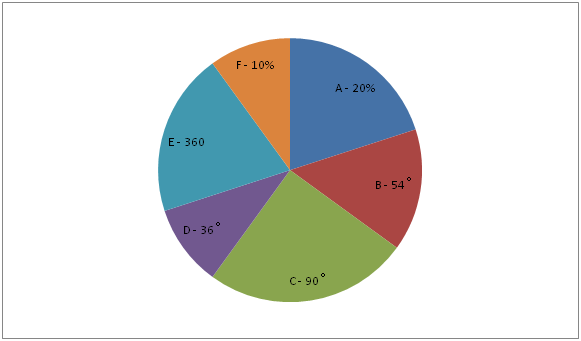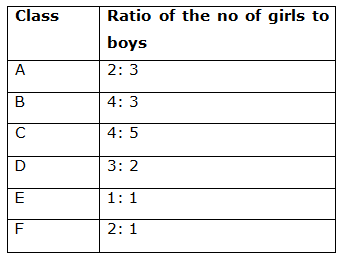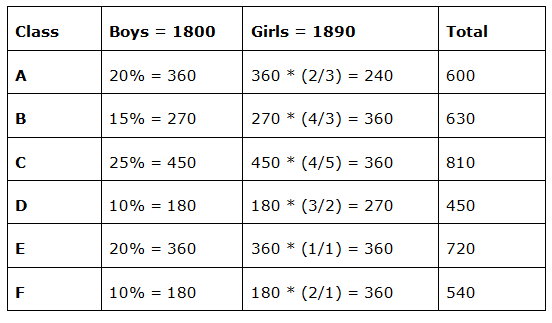# SBI Clerk Pre Quantitative Aptitude (Day-47)

Dear Aspirants, Our IBPS Guide team is providing new series of Quantitative Aptitude Questions for SBI Clerk Prelims 2020 so the aspirants can practice it on a daily basis. These questions are framed by our skilled experts after understanding your needs thoroughly. Aspirants can practice these new series questions daily to familiarize with the exact exam pattern and make your preparation effective.

Start Quiz

Ensure Your Ability Before the Exam – Take SBI Clerk 2020 Prelims Free Mock Test

Directions (1 – 5): Following question contains two equations as I and II. You have to solve both equations and determine the relationship between them and give answer as,

a) x > y

b) x ≥ y

c) x = y or relationship cannot be determined.

d) x < y

e) x ≤ y

1) I) x2 – 11x + 30 = 0

II) y2 – 6y + 5 = 0

2) I) 2x2 + 24x + 54 = 0

II) y2 + 13y + 30 = 0

3) I) x2 – 19x + 84 = 0

II) y2 – 21y + 104 = 0

4) I) x2 – 2x – 48 = 0

II) y2 + 15y + 56 = 0

5) I) x2 = 1156

II) y = 2 * √289

Data Interpretation

Directions (6 – 10): Study the following information carefully and answer the given questions?

The pie chart given below shows the distribution of the number of boys in percentage or in absolute value or in degree in six different classes.6) Find the three – fourth of the number of girls in class F?

a) 180

b) 150

c) 210

d) 190

e) None of these

7) If the ratio of the urban to rural students in class C is 17: 10 and the total urban boys is 240, what is the total number of rural girls in the class C?

a) 120

b) 60

c) 150

d) 90

e) Cannot be determined

8) Total girls in class D is what percentage more/less than the total students in class F?

a) 25%

b) 50%

c) 40%

d) 60%

e) 30%

9) Total girls in all the class together is what percentage more than the total boys in all the class together?

a) 5 ½ %

b) 8 1/3 %

c) 6%

d) 12%

e) 4%

10) What is the ratio of the total boys in class B, C and D together to that of the total girls in class A, E and F?

a) 1: 2

b) 2: 3

c) 15: 16

d) 3: 5

e) None of these

Directions (1-5) :

I) x2 – 11x + 30 = 0

x2 – 6x – 5x + 30 = 0

(x – 5) (x – 6) = 0

x = 5, 6

II) y2 – 6y + 5 = 0

y2 – 5y – y + 5 = 0

(y – 1) (y – 5) = 0

y = 1, 5

Hence, x ≥ y

I) 2x2 + 24x + 54 = 0

2x2 + 18x + 6x + 54 = 0

2x(x + 9) + 6(x + 9) = 0

(2x + 6) (x + 9) = 0

x = -3, -9

II) y2 + 13y + 30 = 0

y2 + 3y + 10y + 30 = 0

(y + 10) (y + 3) = 0

y = -10, -3

Hence, x and y relationship cannot be determined

I) x2 – 19x + 84 = 0

x2 – 12x – 7x + 84 = 0

(x – 7) (x – 12) = 0

x = 7, 12

II) y2 – 21y + 104 = 0

y2 – 8y – 13y + 104 = 0

(y – 13) (y – 8) = 0

y = 13, 8

Relationship between x and y cannot be established.

I) x2 – 2x – 48 = 0

x2 – 8x + 6x – 48 = 0

(x + 6) (x – 8) = 0

x = -6, 8

II) y2 + 15y + 56 = 0

y2 + 7y + 8y + 56 = 0

(y + 7) (y + 8) = 0

y = -7, -8

Hence, x > y

I) x2 = 1156

= > x = ± 34

II) y = 2 * √289

y = 2 * 17 = 34

Hence, x ≤ y

Directions (6 – 10):

Percentage of boys in class A = 20%

Percentage of boys in class B = 54˚ * 100/360 = 15%

Percentage of boys in class C = 90˚ * 100/360 = 25%

Percentage of boys in class D = 36˚ * 100/360 = 10%

Percentage of boys in class F = 10%

Percentage of boys in class E

= 100% – (20% + 15% + 25% + 10% + 10%)

= 100% – 80% = 20%

From the graph,

Number of boys in class E = 20% of total males = 360

Total boys = 360/20 * 100 = 1800Three fourth of the number of girls in class F = ¾ * 360 = 270

Total Urban students in class C = 810 * 17/27 = 510

Total Rural students in class C = 810 * 10/27 = 300

Total Urban girls in class C = 510 – 240 = 270

Total Rural girls in class C = 360 – 270 = 90

Required percentage = (540 – 270)/540 * 100

= 270/540 * 100 = 50% less

Required percentage = (1950 – 1800)/1800 * 100

= 1500/1800 * 100

= 8 1/3 %